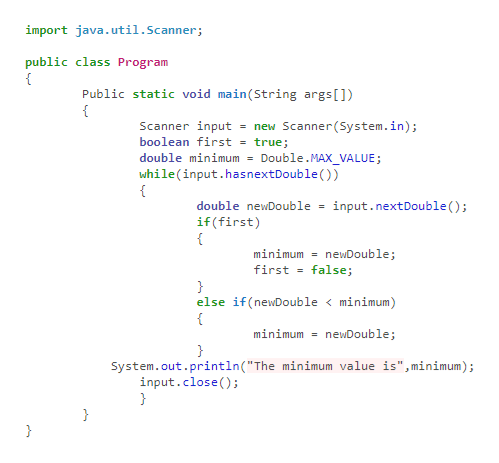# Translate the following pseudocode for finding the minimum value from a set of inputs into a Java program.Set a Boolean variable “first” to true. While another value has been read successfully If first is true Set the minimum to the value just read Set first to false Else if the value is less than the minimum Set the minimum to the value Print the minimum

Question
54 views

Translate the following pseudocode for finding the minimum value from a set of inputs into a Java program.
Set a Boolean variable “first” to true.
While another value has been read successfully
If first is true
Set the minimum to the value just read
Set first to false
Else if the value is less than the minimum
Set the minimum to the value
Print the minimum

check_circle

Java program to find the minimum value

import java.util.Scanner;

public class Program

{

Public static void main(String args[])

{

Scanner input = new Scanner(System.in);

boolean first = true;

double minimum = Double.MAX_VALUE;

while(input.hasnextDouble())

{

double newDouble = input.nextDouble();

if(first)

{

minimum = newDouble;

...help_outlineImage Transcriptioncloseimport java.util.Scanner; public class Program Public static void main(String args[]) Scanner input = new Scanner(System.in) boolean first= true; double minimum = Double.MAX_VALUE; while (input.hasnextDouble () ) input.nextDouble ( ) ; double newDouble if (first) minimum = newDouble; false; first } else if (newDouble < minimum) minimum = neWDouble; } System.out.println("The minimum value is", minimum); input.close() } } } fullscreen

### Want to see the full answer?

See Solution

#### Want to see this answer and more?

Solutions are written by subject experts who are available 24/7. Questions are typically answered within 1 hour.*

See Solution
*Response times may vary by subject and question.
Tagged in

### Computer Science